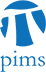## Approximating the Solution to a Static Hamilton-Jacobi Equation in a Single Monotone Pass

• Date: 03/30/2010
Lecturer(s):
Ken Alton (UBC)
Location:

University of British Columbia

Description:

In the context of optimal control, the Hamilton-Jacobi Partial Differential Equation (HJ PDE) is a continuous analogue to the discrete Bellman dynamic-programming equation. Both of these equations satisfy a causal property: the solution value at a state is independent of equal or greater solution values. Dijkstra's algorithm is a dynamic-programming method that exploits this causal property to compute the minimal path costs from a source node to all nodes in a discrete graph in a single pass. The Fast Marching Method (FMM) is an analogous single-pass method for approximating the continuous solution to the Eikonal equation, an HJ PDE for which the speed of motion is the same in all directions. We present a generalization of FMM, a single-pass method that approximates the solution to a static HJ PDE for which the speed of motion may depend on the direction of travel. We use examples drawn from robot path planning and seismology to demonstrate the benefits over competing methods.

Schedule:

12:30 - 2:00pm, WMAX 216.